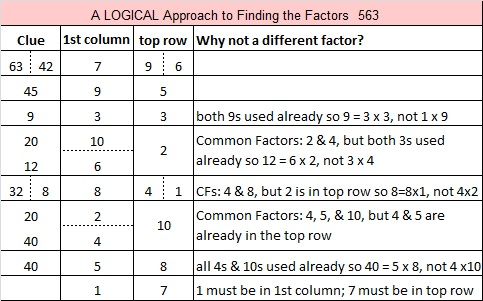Today is a Good Day to Review Proof by Induction

0² = 0
1²  = 1

Does that pattern hold for all natural numbers? Could we claim that n²  = n?

Yes, we can, and I’ve written a proof to prove it! The proof uses a valuable concept in mathematics called induction. I remember being introduced to proofs by induction when I was in Junior High. Nowadays, if it is not part of Common Core, it wouldn’t be taught much anymore. Nevertheless, I will use it here to prove that n² = n.Using a similar proof, we can also prove that n³ = n, n⁴ = n, n⁵ = n, n⁶ = n, and so forth!

Today is the perfect day to review how to use proof by induction so try your hand at proving at least one of those mathematical statements on your own. Use the same steps in my example: prove true for n=1, assume true for n = k, prove true for k + 1, write your conclusion. then have a very Happy April Fools’ Day, Everyone!

Today is also a very good day to review that (x + y)² = x² +2xy + y²  and NOT x²  + y², a very common error students make. Confession: I remember making that exact error in high school when I definitely should have known better. Using induction to prove something in mathematics is a valid technique, but if you use invalid equations like
(x + y)³ = x³ + y³, you will make invalid conclusions. Thus, today might also be a good day to review the binomial theorem and Pascal’s triangle. (Pascal’s triangle has numbers in its interior, not just 1’s going down the sides, after all.)

My post today was inspired by a post written by Sara Van Der Werf titled Why I’ve Started Teaching the FOIL Method Again. In her post, she not only plays a great April Fools’ joke on her readers, but she explains a tried and true way to multiply binomials and other polynomials.

I read her post exactly one year ago today, and since then, I have been waiting for April Fools’ Day to roll around again so that I could share this post with you. It is my hope that you will enjoy my little prank and learn a little mathematics from it as well.

1366 Fractions Acting Improperly

In elementary school, we learned about improper fractions. Should we call them that? Is it even possible for any kind of number to be IMPROPER? They are simply fractions greater than one. I’ve recently heard the term fraction form used, and ever since I’ve made a point of saying that fractions greater than one are in fraction form.On Twitter, I’ve found a few people who also don’t like using the word improper to describe any fraction.

This first tweet has a link explaining why it is improper to use the term improper fraction:

I hope that you will consider not labeling any fraction as improper, as well!

Now I’ll write a little bit about the number 1366:

• 1366 is a composite number.
• Prime factorization: 1366 = 2 × 683
• The exponents in the prime factorization are 1 and 1. Adding one to each and multiplying we get (1 + 1)(1 + 1) = 2 × 2 = 4. Therefore 1366 has exactly 4 factors.
• Factors of 1366: 1, 2, 683, 1366
• Factor pairs: 1366 = 1 × 1366 or 2 × 683
• 1366 has no square factors that allow its square root to be simplified. √1366 ≈ 36.959441366 is also the sum of the twenty-six prime number from 5 to 107. Do you know what all those prime numbers are?

1350 Logic is at the Heart of This Puzzle

By simply changing two clues of that recently published puzzle that I rejected, I was able to create a love-ly puzzle that can be solved entirely by logic. Can you figure out where to put the numbers from 1 to 12 in each of the four outlined areas that divide the puzzle into four equal sections? If you can, my heart might just skip a beat!

Now I’ll tell you a few things about the number 1350:

• 1350 is a composite number.
• Prime factorization: 1350 = 2 × 3 × 3 × 3 × 5 × 5, which can be written 1350 = 2 × 3³ × 5²
• The exponents in the prime factorization are 1, 3 and 2. Adding one to each and multiplying we get (1 + 1)(3 + 1)(2 + 1) = 2 × 4 × 3 = 24. Therefore 1350 has exactly 24 factors.
• Factors of 1350: 1, 2, 3, 5, 6, 9, 10, 15, 18, 25, 27, 30, 45, 50, 54, 75, 90, 135, 150, 225, 270, 450, 675, 1350
• Factor pairs: 1350 = 1 × 1350, 2 × 675, 3 × 450, 5 × 270, 6 × 225, 9 × 150, 10 × 135, 15 × 90, 18 × 75, 25 × 54, 27 × 50 or 30 × 45
• Taking the factor pair with the largest square number factor, we get √1350 = (√225)(√6) = 15√6 ≈ 36.74235

1350 is the sum of consecutive prime numbers two ways:
It is the sum of the fourteen prime numbers from 67 to 131, and
673 + 677 = 1350

1350 is the hypotenuse of two Pythagorean triples:
810-1080-1350 which is (3-4-5) times 270
378-1296-1350 which is (7-24-25) times 54

1350 is also the 20th nonagonal number because 20(7 · 20 – 5)/2 = 1350

Facts about and Factors of 2019

Here’s a countdown you can use to ring in the New Year: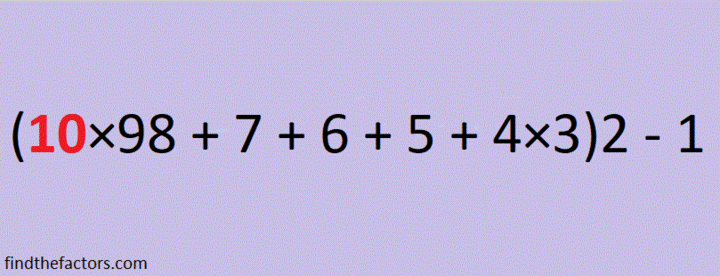make science GIFs like this at MakeaGif

2019 is the sum of consecutive numbers three different ways:
1009 + 1010 = 2019
672 + 673 + 674 = 2019
334 + 335 + 336 + 337 + 338 + 339 = 2019

There is one way that 2019 is the sum of consecutive odd numbers:
671 + 673 + 675 = 2019

2019 is the difference of two squares two different ways:
338² – 335² = 2019
1010² – 1009² = 2019

2019 is the sum of three squares nine different ways:
43² + 13² + 1² = 2019
43² + 11² + 7² = 2019
41² + 17² + 7² = 2019
41² + 13² + 13² = 2019
37² + 25² + 5² = 2019
37² + 23² + 11² = 2019
37² + 19² + 17² = 2019
35² + 25² + 13² = 2019
31² + 23² + 23² = 2019

2019 is the hypotenuse of a Pythagorean triple:
1155-1656-2019 so 1155² + 1656² = 2019²

2¹⁰ + 2⁹ + 2⁸ + 2⁷ + 2⁶ + 2⁵ + 2¹ + 2⁰ = 2019

2019 is a palindrome in a couple of bases:
It’s 5B5 in BASE 19 (B is 11 base 10) because 5(19²) + 11(19) + 5(1) = 2019,
and 3C3 in BASE 24 (C is 12 base 10) because 3(24²) + 12(24) + 3(1) = 2019

Every year has factors that often catch people by surprise. Today I would like to give you my predictions for the factors of 2019:
2019 will have four positive factors: 1, 3, 673, and 2019
However, 2019 will also have four negative factors: -1, -3, -673, and -2019

Which factors, positive or negative, will be your focus in the coming year?

Finally, I’ll share some mathematics-related 2019 and New Year tweets that I’ve seen on twitter. Some of these tweets have links that contain even more facts about the number 2019.

825 Quarters Make Dividing by 25 Easy

Numbers ending in 00, 25, 50, and 75 can be divided evenly by 25. How much is 825 divided by 25? That quotient is the same as the answer to “how many quarters are in \$8.25?” (A quarter is ¼ of a dollar and is written .25 or 25¢.)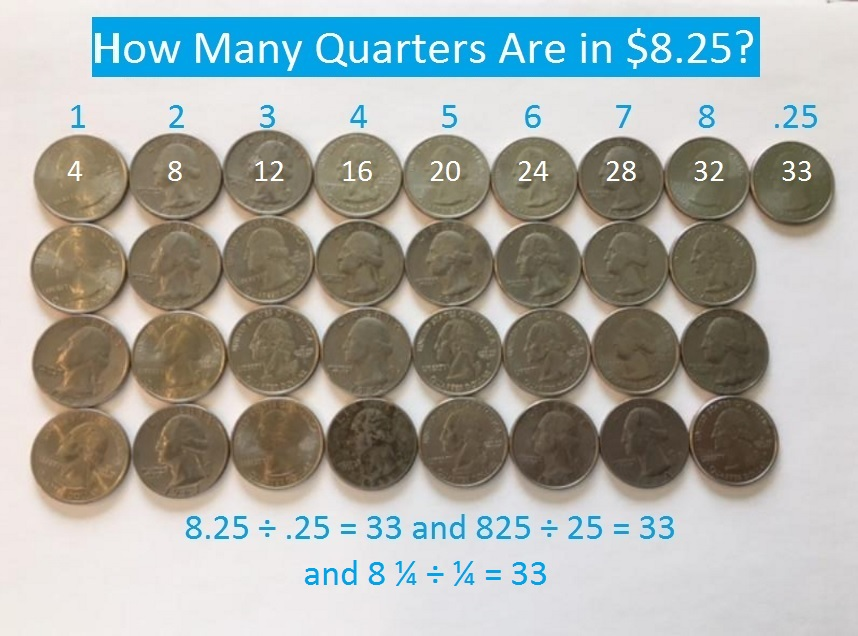You probably could visualize the answer in your head even if I hadn’t included a picture! That’s why I often ask kids the how-many-quarters question when they are stumped dividing something by 25 . It seems that kids are always able to give the quotient after that question. Notice that “8.25 ÷ .25 =” and  “8 ¼ ÷ ¼ =” have the same answer, too. You can also ask that how-many-quarters question to find the answer when something is divided by .25 or ¼.

It would almost be as easy to divide \$8.26 or \$8.39 by 25. The quotient would be the same as the problem above but with some loose change becoming the remainder. Using money for division problems could even help kids better understand dividends, divisors, quotients, and remainders.

Here’s an example of a how-many-quarters type question that will help you divide by 75, .75 or ¾.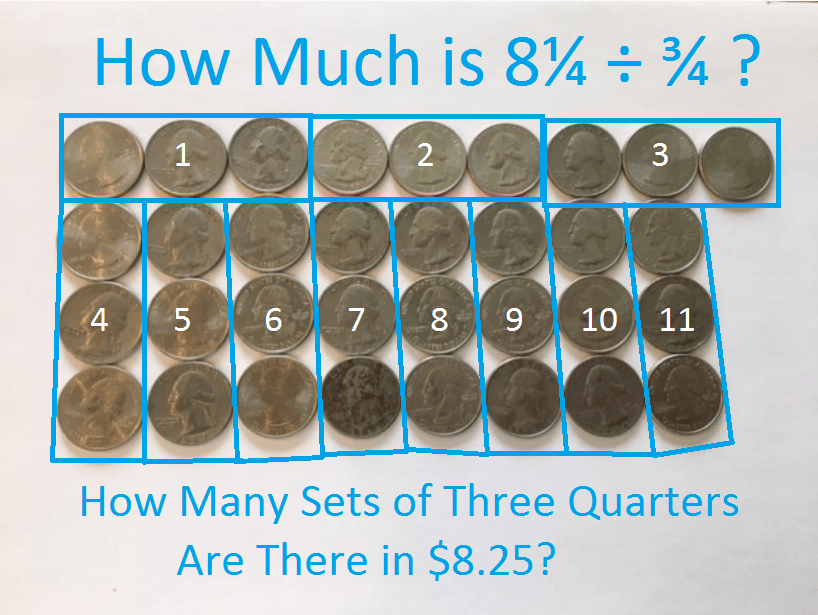We can count and see that there are 11 sets of 3 quarters in \$8.25. That means that \$8.25 ÷ .75 is 11. It also means that 8¼ ÷ ¾ = 11.

Dividing by fractions can be a very abstract concept for students, and they may ask questions like, “What does 8¼ ÷ 1¼ even mean?” Again quarters come to the rescue! 5 quarters can be so much more friendly than 1¼ is. Shorthand for 5 quarters is the fraction, 5/4. Since they have the same denominators, dividing 8¼ by 1¼ is the same as dividing 33 by 5:Kids think money is more fun than math, but money is just a subset of mathematics which is full of lots of other fun topics, too. Here are a couple of ways other educators have used money to teach a math topic.

Jen of Beyond Tradit’l Math shared her way to teach subtracting decimals using money. Her method will surely captivate any child who tries it and even make regrouping fun:

Robert Kaplinsky uses several very short videos to keep students engaged without them actually touching any money. Check out the replies, too. Paula Beardell Krieg’s excellent \$1.00 art project is there, and that would be fun for anyone 2nd grade or older to do:

Now back to the number 825.

Clearly 825 has to be divisible by both 5 AND 3 in order for (821, 823, 827, 829) to be the fourth prime decade, which it is.

• The last digit of 825 is 5, so it is divisible by 5.
• 8 + 2 + 5 = 15, a multiple of 3, so 825 is divisible by 3.
• 8 – 2 + 5 = 11, so 825 is divisible by 11.

All numbers ending in 00, 25, 50, or 75 can have their square roots simplified. If you were trying to simplify √825, you could visualize quarters in your mind to easily divide 825 by 25. Then √825 = (√25)(√33) = 5√33

Here is 825’s factoring information:

• 825 is a composite number.
• Prime factorization: 825 = 3 × 5 × 5 × 11, which can be written 825 = 3 × 5² × 11
• The exponents in the prime factorization are 1, 2, and 1. Adding one to each and multiplying we get (1 + 1)(2 + 1)(1 + 1) = 2 × 3 × 2 = 12. Therefore 825 has exactly 12 factors.
• Factors of 825: 1, 3, 5, 11, 15, 25, 33, 55, 75, 165, 275, 825
• Factor pairs: 825 = 1 × 825, 3 × 275, 5 × 165, 11 × 75, 15 × 55, or 25 × 33
• Taking the factor pair with the largest square number factor, we get √825 = (√25)(√33) = 5√33 ≈ 28.72281810 Stick and StonePrint the puzzles or type the solution on this excel file: 10-factors 807-814One of my education professors taught that you can teach any concept with a picture book.

I recently read the book, Stick and Stone, to a class of 6th graders. Yes, 6th graders. You can get away with reading something way below grade level if you tell them before you start reading that you will use the book to introduce them to something that is definitely NOT below grade level. The first few pages of the book are shared by its publisher here:

As you can see, those first few pages equate stone as a zero and stick as a lonely number one.

The middle part of the book teaches about synergizing, working together to make life good and helping each other through tough times.

By the end of the book Stick and Stone know how to work very well together, “Stick, Stone. Together again. Stick, Stone. A perfect ten.”

The book pretty much ends there, but making a perfect ten is only the beginning of what these two characters can do together. I used this book to teach the class not only about getting along and working together, but also about base 2, or binary as it is also called. Every counting number we know can be represented by using just 1’s and 0’s. I wrote on the board the numbers from 1 to 16 and represented the first few of those numbers in base 2. Then I invited class members to come up with how to write the rest of the numbers in base 2. Some students caught on immediately while the others were able to learn how to do it by watching their classmates and listening to them. Eventually with at least 12 different student’s inputs, we came up with a chart that looked something like this:Notice that the numbers from 9 to 15 are just 1000 plus the numbers directly across from them in the first column.

Some of the sixth grade students had already heard of binary, so I showed them a little more about base 2: I wrote a bunch of 1’s and 0’s “off the top of my head” onto the board and added the headings to show place values: 1’s place, 2’s place, etc.Then I told them to sum up the place values that contained a one: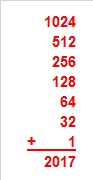Stick and Stone are the main two characters, but the book has one other character, Pinecone. At first Pinecone bullied Stone, but after Stick stood up to him, the three of them were eventually able to become friends. You might enjoy finding out more about Pinecone by listening to Sean Anderson read the entire book to his children, one of which seems to really enjoy numbers.

If you used a unique symbol to represent Pinecone, it could look like a 2. Then you also could use the symbols 0, 1, and 2 to represent every counting number in base 3. That’s another concept the picture book Stick and Stone could be used to introduce!

To make a chart for base 3, start with these 3 columns of numbers with 3 numbers in each: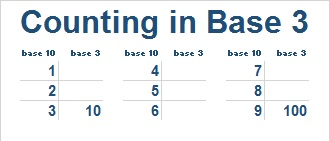Since this is base 3, where should 10 and 100 go? The bottom of the first column and the bottom of the third column both MUST look like a power of 10. The rest of the chart is easy to fill out. Notice the 1 and 2 look exactly the same in base 10 and base 3. Also since 4 = 3 + 1, 5 = 3 + 2, and 6 = 3 + 3, we can easily fill in the 2nd column. Two more addition facts will finish the third column: 7 = 6 + 1, and 8 = 6 + 2.Now add what you learned about 4, 5, 6, 7, 8, and 9 to column 1 and put the numbers 10 – 18 in the base 10 second column and numbers 19 – 27 in the base 10 third column. Again the bottom of the first column and the bottom of the third column both MUST look like a power of 10, so we now know where to put 1000.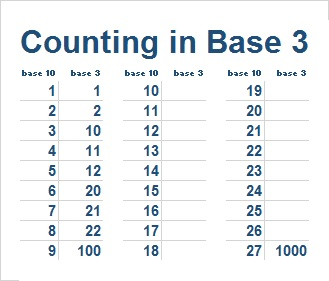To fill in the rest of the chart simply add 100 to the base 3 numbers in column 1 to get the the base 3 numbers in column 2. Then add 200 to the base 3 numbers in column 1 to get the remaining base 3 numbers in column 3.You could do this process again to determine the first 81 counting numbers in base 3 with 81 being represented by 10000.

For base 4, you could do something similar with 4 columns. However, for counting in bases 4, 5, 6, 7, 8, and 9 I would suggest using the very versatile hundred chart. You can give instructions without even mentioning the concept of differing bases. For example, cross out every number on the hundred chart that has 7, 8, or 9 as one or more of its digits. Can you tell even before you get started how many numbers will get crossed out? (100 – 7²) What pattern do the cross-outs make? If you arrange the remaining numbers in order from smallest to largest, then you will have the first 49 numbers represented in base 7. With a minimal amount of cutting and taping you could have a “hundred” chart in base 7. Easy peasy.

This excel file not only has several puzzles, including today’s, but also a hundred chart and even a thousand chart because I know some of you might want to play with 3-digit numbers, too.

Now let me tell you a little bit about the number 810:

• 810 is a composite number.
• Prime factorization: 810 = 2 x 3 x 3 x 3 x 3 x 5, which can be written 810 = 2 × 3⁴ × 5
• The exponents in the prime factorization are 1, 4 and 1. Adding one to each and multiplying we get (1 + 1)(4 + 1)(1 + 1) = 2 x 5 x 2 = 20. Therefore 810 has exactly 20 factors.
• Factors of 810: 1, 2, 3, 5, 6, 9, 10, 15, 18, 27, 30, 45, 54, 81, 90, 135, 162, 270, 405, 810
• Factor pairs: 810 = 1 x 810, 2 x 405, 3 x 270, 5 x 162, 6 x 135, 9 x 90, 10 x 81, 15 x 54, 18 x 45 or 27 x 30
• Taking the factor pair with the largest square number factor, we get √810 = (√81)(√10) = 9√10 ≈ 28.4604989.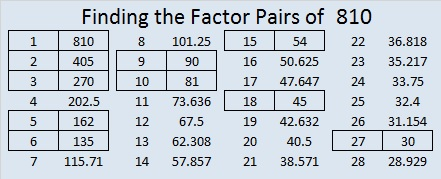Since 810 has so many factors, it has MANY possible factor trees. If most people made a factor tree for 810, they would probably start with 81 × 10 or 9 x 90. NOT ME! Here are two less-often-used factor trees for 810:Finally, here is an easy way to express 810 is in a different base:

1. Make a cake in which you divide 810 by the base number repeatedly, keeping track of the remainders, including zero, as you go.
2. Keep dividing until the number at the top of the cake is 0.
3. List the remainders in order from top to bottom and indicate the base you used to do the division.
4. This method is illustrated for BASE 2 and BASE 3 below:That’s all pretty good work for a stone, a stick, and a pine cone!

By the way, using that method will also produce the following results:

• 810 is 30222 BASE 4
• 810 is 11220 BASE 5
• 810 is 3430 BASE 6 and so forth.

And just so you’ll know, 810 is the sum of consecutive primes 401 and 409.

807 and Level 1

What can I say about the number 807?

807 is palindrome 151 in BASE 26 because 1(26²) + 5(26) + 1(1) = 807.

Anything else? Well, I can figure out a few other things because 807’s has two prime factors, 3 and 269:

We can write ANY number (unless it’s a power of 2) as the sum of consecutive numbers in at least one way. 807 has three different ways to do that:

• 403 + 404 = 807 because 807 isn’t divisible by 2.
• 268 + 269 + 270 = 807 because it is divisible by 3.
• 132 + 133 + 134 + 135 + 136 + 137 = 807 since it is divisible by 3 but not by 6.

I know that one of 807’s factors, 269, is a hypotenuse of a Pythagorean triple, so 807 is also. Thus. . .

• (3·69)² + (3·260)² = (3·269)², or in other words, 207² + 780² = 807²

Since 807 has two odd sets of factor pairs, I know that 807 can be written as the difference of two squares two different ways:

• 136² – 133² = 807
• 404² – 403² = 807

I don’t usually do this, but today’s puzzle has something in common with 807. Can you tell what it is?Print the puzzles or type the solution on this excel file: 10-factors 807-814

• 807 is a composite number.
• Prime factorization: 807 = 3 x 269
• The exponents in the prime factorization are 1 and 1. Adding one to each and multiplying we get (1 + 1)(1 + 1) = 2 x 2 = 4. Therefore 807 has exactly 4 factors.
• Factors of 807: 1, 3, 269, 807
• Factor pairs: 807 = 1 x 807 or 3 x 269
• 807 has no square factors that allow its square root to be simplified. √807 ≈ 28.4077454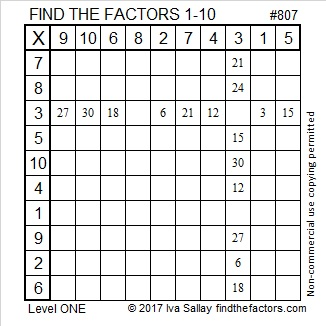572 and Level 1

72 can be evenly divided by 4 so 572 is divisible by 4.

5 – 7 + 2 = 0, which can be evenly divided by 11, so 11 is a factor of 572.

572 is the hypotenuse of the Pythagorean triple 220-528-572. Which factor of 572 is the greatest common factor of those three numbers?Print the puzzles or type the solution on this excel file: 10 Factors 2015-08-03

—————————————————————————————————

• 572 is a composite number.
• Prime factorization: 572 = 2 x 2 x 11 x 13, which can be written 572 = (2^2) x 11 x 13
• The exponents in the prime factorization are 2, 1, and 1. Adding one to each and multiplying we get (2 + 1)(1 + 1)(1 + 1) = 3 x 2 x 2 = 12. Therefore 572 has exactly 12 factors.
• Factors of 572: 1, 2, 4, 11, 13, 22, 26, 44, 52, 143, 286, 572
• Factor pairs: 572 = 1 x 572, 2 x 286, 4 x 143, 11 x 52, 13 x 44, or 22 x 26
• Taking the factor pair with the largest square number factor, we get √572 = (√4)(√143) = 2√143 ≈ 23.91652—————————————————————————————————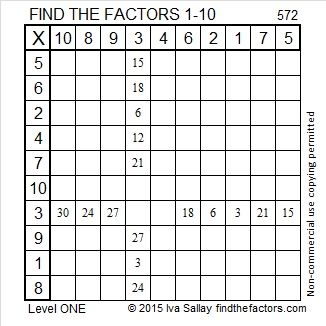564 and Level 6

Consecutive primes 281 and 283 add up to 564.

564 is made from three consecutive numbers so it can be evenly divided by 3. If the middle number is divisible by 3, then a number made from three consecutive numbers will also be divisible by 9. Is 564 divisible by 9? Why or why not?Print the puzzles or type the solution on this excel file: 10 Factors 2015-07-20

—————————————————————————————————

• 564 is a composite number.
• Prime factorization: 564 = 2 x 2 x 3 x 47, which can be written 564 = (2^2) x 3 x 47
• The exponents in the prime factorization are 2, 1, and 1. Adding one to each and multiplying we get (2 + 1)(1 + 1)(1 + 1) = 3 x 2 x 2 = 12. Therefore 564 has exactly 12 factors.
• Factors of 564: 1, 2, 3, 4, 6, 12, 47, 94, 141, 188, 282, 564
• Factor pairs: 564 = 1 x 564, 2 x 282, 3 x 188, 4 x 141, 6 x 94, or 12 x 47
• Taking the factor pair with the largest square number factor, we get √564 = (√4)(√141) = 2√141 ≈ 23.74868—————————————————————————————————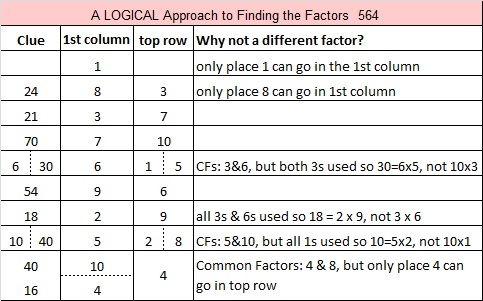563 and Level 5

563 is prime, and it is the sum of all the prime numbers from 5 to 67.Print the puzzles or type the solution on this excel file: 10 Factors 2015-07-20

—————————————————————————————————

• 563 is a prime number.
• Prime factorization: 563 is prime.
• The exponent of prime number 563 is 1. Adding 1 to that exponent we get (1 + 1) = 2. Therefore 563 has exactly 2 factors.
• Factors of 563: 1, 563
• Factor pairs: 563 = 1 x 563
• 563 has no square factors that allow its square root to be simplified. √563 ≈ 23.727621How do we know that 563 is a prime number? If 563 were not a prime number, then it would be divisible by at least one prime number less than or equal to √563 ≈ 23.7. Since 563 cannot be divided evenly by 2, 3, 5, 7, 11, 13, 17, 19, or 23, we know that 563 is a prime number.

—————————————————————————————————